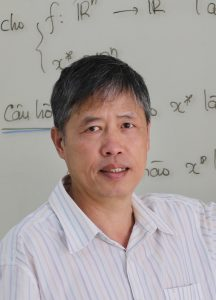# A.PROF. DR. PHAM TIEN SONDr. Phạm Tiến Sơn was born in 1964 in Hai Phong, Vietnam. He graduated from University of Da Lat in 1985 and then received his PhD degree from the Institute of Mathematics, the Vietnam Academy of Science and Technology, in 2005 in the field of Geometry and Topology. He has joined University of Da Lat since 1985 as a lecturer. He is also a member of Scientific Council of Vietnam Institute for Advanced Studies in Mathematics from 2018 to 2021. His research interests focus on geometry, topology and, in particular, singularities at infinity of polynomial mappings. Recently, he studied on semialgebraic optimization problems. He, together with his colleagues, has published one monograph and more than 50 research papers in international peer-reviewed journals.

Work

Gue Myung Lee and Tien Son Pham, 2017. Generic properties for semialgebraic programs. SIAM Journal on Optimization, Vol. 27, No. 3, 2061–2084

In this paper we study genericity for the class of semialgebraic optimization problems with equality and inequality constraints, in which every problem of the class is obtained by linear perturbations of the objective function, while the “core” objective function and the constraint functions are kept fixed. The meaning of genericity is that a given property holds in a nonempty, Zariski open subset of the space of all problems; this implies that one can assume that the property holds after a general enough perturbation of the input data.

There are two main contributions. The first result states that almost all problems in the class are such that (i) the restriction of the objective function on the constraint set is a stratified Morse function, is coercive and regular at infinity; (ii) there is a unique optimal solution, lying on a unique active manifold, at which the strict complementarity and second order sufficiency conditions, the quadratic growth condition, and the Hölder type global error bound hold, and (iii) all minimizing sequences converge. Furthermore, the active manifold is constant, and the optimal solution and the optimal value function depend analytically under local perturbations of the objective function.

The second main result states that one may build a sequence of semidefinite programming (SDP) problems whose solutions give rise to a sequence of points converging to the optimal solution of the original problem in finitely many steps. It should be note that an SDP problem is the natural generalization of linear programming over the cone of positive semidefinite matrices and plays a universal role in polynomial optimization thanks to the Lasserre method.

It is worth emphasizing that the results of this paper hold globally and we do not require the problem to be convex or the constraint set to be compact.

The ideas for our investigation come from Theory of Singularities. On the other hand, the main tools come from Semialgebraic Geometry.